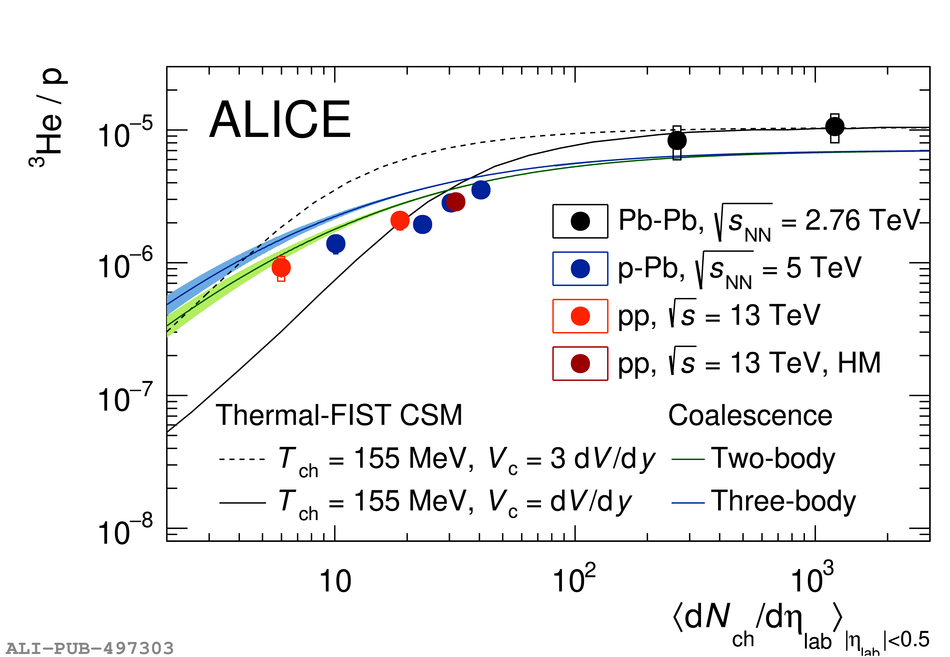# Figure 10

 Ratio between the \pt-integrated yields of nuclei and protons as a function of multiplicity for (anti)deuterons (a) and (anti)helions (b). Measurements are performed in HM pp collisions at $\sqrt{s}~=~13$~TeV, in MB pp collisions at $\sqrt{s}~=~13$~TeV~ and at $\sqrt{s}~=~7$~TeV~, in p--Pb collisions at $\snn~=~5.02$~TeV~, and in Pb--Pb collisions at $\snn~=~2.76$~TeV~. Vertical bars and boxes represent statistical and systematic uncertainties, respectively. The two black lines are the theoretical predictions of the Thermal-FIST CSM~ for two sizes of the correlation volume $V_{\mathrm{C}}$. For (anti)deuterons, the green line represents the expectation from a coalescence model~. For (anti)helion, the blue and green lines represent the expectations from a two-body and three-body coalescence model, respectively~.